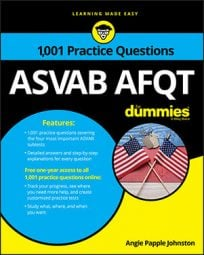##### ASVAB AFQTOn the Mathematics Knowledge subtest on the ASVAB, questions involving three-dimensional figures may jump off the screen at any time. You should be ready for them by memorizing the volume formulas for the most common 3D figures, like spheres, cubes, and pyramids.

## Practice questions

1. Find the volume of the right square pyramid:

A. 450 cm3 B. 500 cm3 C. 1,500 cm3 D. 150 cm3

2. Find the volume:

A. 960 mm3 B. 480 mm3 C. 120 mm3 D. 840 mm3

1. The correct answer is Choice (B).

The formula for the volume of a pyramid is

where B is the area of the pyramid's base and h is the pyramid's height. You're dealing with a right square pyramid, so the base is a square. Because a square has an area of A = s2 (where s is the length of one side of the base), you can write the formula as

Replace the variables with the numbers you know:

2. The correct answer is Choice (A).

The formula for volume of a right rectangular prism is V = lwh, with l representing length, w representing width, and h representing height: